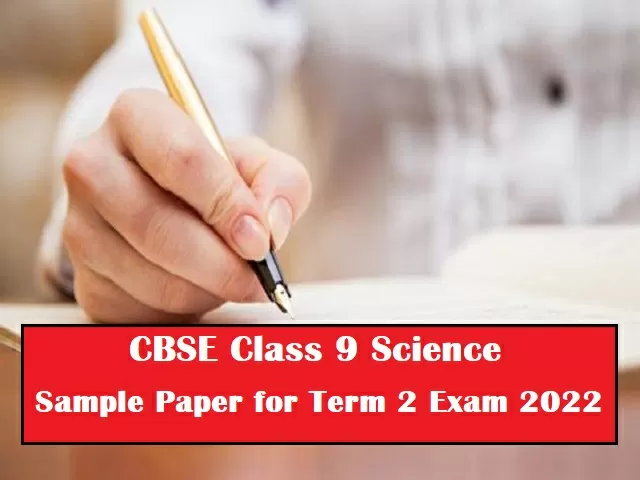# CBSE Class 9 Science Sample Paper with Solution for Term 2 Exam 2022 (By Exam Experts)

## CBSE Class 9 Science Sample Paper for Term 2 Exam 2022 is provided here to practice important questions for the upcoming exam. Students can also check answers to all questions for reference and quick revision.CBSE Class 9 Science Sample Paper for Term 2 Exam 2022

CBSE Class 9 Term 2 Exam 2022: CBSE Class 9 students can now increase their score in their Science Term 2 paper by practicing the sample paper provided below. The CBSE Class 9 Science Term 2 Sample Paper 2022 which is provided below, is compiled by experienced teachers. This sample paper is prepared entirely according to the latest paper pattern following the CBSE Class 9 Science Term 2 Syllabus. Marking scheme of the sample paper is also provided at the end of the paper to help students cross check their answers and correct their mistakes if any.

Also, Check

Check CBSE Class 9 Science Sample Paper for Term 2 Exam 2022 below:

General instructions:

1. All questions are compulsory.
2. The question paper has three sections.
3. Section A consists of seven questions of 2 marks each.
4. Section B consists of six questions of 3 marks each.
5. Section C consists of two case-study based questions of 4 marks each.
6. Internal choice is given in some questions. The child has to attempt any one of the alternatives.

SECTION - A

1. Under which of the following conditions are you most likely to fall sick? (2)

(a) When you are taking examinations.

(b) When you have travelled by bus for two days.

(c) When your friend is suffering from measles. Why?

2. Write the full form of AlDS. Name the virus responsible for its cause. (1+1 = 2)

3. If number of electrons in an atom is 8 and number of protons is also 8,then (1+1=2)

(i) What is the atomic number of the atom?

(ii)What is the charge on the atom?

4. What is the magnitude of the gravitational force between the earth and a 1 kg object on its surface? (Mass of the earth is 6 x 1024 kg & Radius of the earth is 6.4 x 106 m) (2)

Or

Derive the expression for universal law of gravitation.

5. (i) When do we say that work is done? (1+1=2)

(ii) Give an example of zero work done.

6. State giving reasons whether the following statements are correct or incorrect. (1+1=2)

(i) Health is only the absence of any physical disease.

(ii) Children need not be immunized.

Or

Why antibiotics are ineffective against viruses?

7. Classify the following diseases as infectious or non-infectious:(1/2x4=2)

(a) Tuberculosis

(b) Malaria

(c) High blood pressure

(d) Chickenpox

SECTION - B

8. Write any three postulates of Dalton's atomic theory. (3)

Or

(a) What do you mean by electronic configuration of an atom? (1+2=3)

(b) Write the electronic configuration for Magnesium and Chlorine atom.

9. (a) What is kinetic energy of a body? (1+2=3)

(b) Derive the expression for kinetic energy.

10. (a) Write one point of difference between isotopes and isobars. (1+2=3)

(b) Write any two applications/uses of isotopes.

11. In a gold foil experiment: (1x3=3)

(a) Why did many alpha particles pass through the gold foil undeflected?

(b) Why did few alpha particles deflect through small angles?

(c) Why did few alpha particles, after striking the gold foil, retrace their path?

Or

Write any three postulates of Bohr theory.

12. (a) Define 1 joule of work. (1+2=3)

(b) Certain force acting on a 25 kg mass changes its velocity from 6 m/s to 4 m/s. Calculate the work done by the body.

13. Why metals are electropositive whereas non-metals are electronegative in nature? (3)

SECTION - C

This section has 2 case-study based questions (14 & 15). Each case is followed by three sub-questions (a, b & c). Part a & b are compulsory. However, an internal choice is provided in part c.

According to Universal law of gravitation, the force of gravitation between two particles/bodies is directly propotional to product of their masses and is inversely proportional to the square of the distance between them. G is a universal constant as it value remains the same throughout the universe.

14(a) What is the SI unit of G? (1)

14(b) How does the force of gravitation between two objects change when the distance between them is reduced to half? (1)

14(c) Calculate the force of gravitation between the earth and the sun, given that mass of the earth is 6 x 1024 kg and of the sun is 2 x 1030 kg. The average distance between the two is 1.5 x 1011 m. (2)

Or

State any two importance of universal law of gravitation.

An atom is the smallest particle of matter. There are three sub-atomic particles of an atom i.e., electrons, protons and neutrons. The number of electrons gives the idea of the atomic number of an atom. The tendency to gain or lose electrons decides the charge on an atom.

15(a) What is the charge present on Sulphur atom? (1)

15(b) What would be the valency, if the atom has 3 protons and 4 neutrons in its nucleus? (1)

15(c) (i) What is atomicity? (2)

(ii) How many atoms are present in PO43 ion and NO3 ion?

Or

What makes an atom electrically neutral?

Check answers and marking scheme of the sample paper below: